# （图） – Dijkstra和Floyd算法

1.Dijkstra算法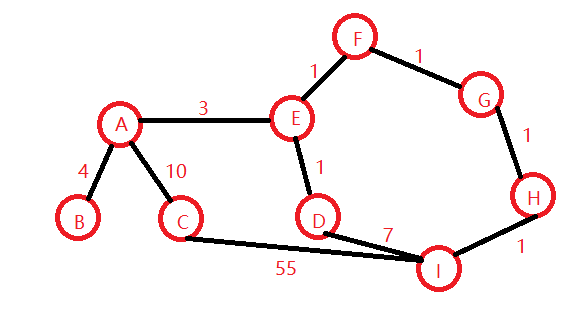索引值 0 1 2 3 4 5 6 7 8 A: 0 0 4 10 ∞ 3 ∞ ∞ ∞ ∞ B: 1 4 0 ∞ ∞ ∞ ∞ ∞ ∞ ∞ C: 2 10 ∞ 0 ∞ ∞ ∞ ∞ ∞ 55 D: 3 ∞ ∞ ∞ 0 1 ∞ ∞ ∞ 7 E: 4 3 ∞ ∞ 1 0 1 ∞ ∞ ∞ F: 5 ∞ ∞ ∞ ∞ 1 0 1 ∞ ∞ G: 6 ∞ ∞ ∞ ∞ ∞ 1 0 1 ∞ H: 7 ∞ ∞ ∞ ∞ ∞ ∞ 1 0 1 I: 8 ∞ ∞ ∞ 55 7 ∞ ∞ 1 0

1.1 开始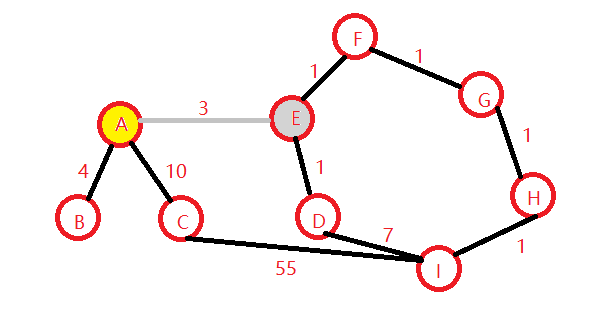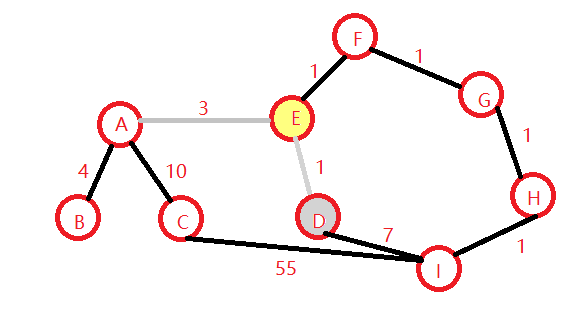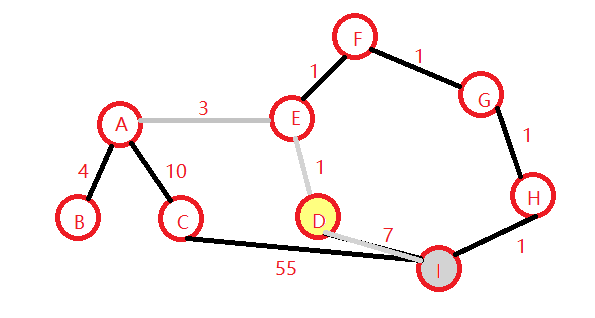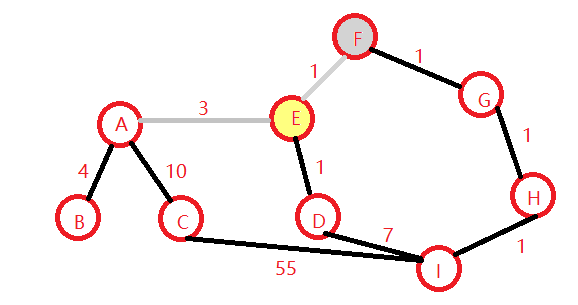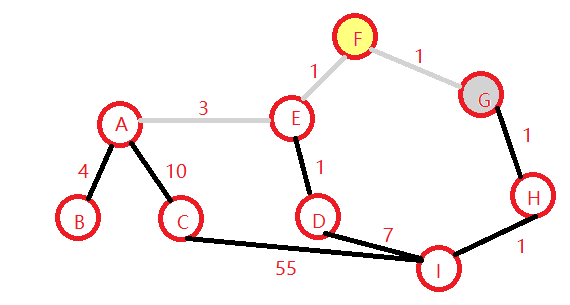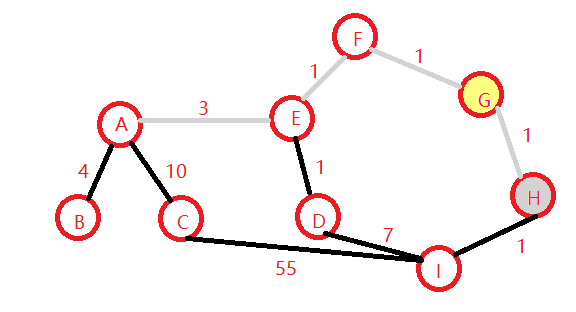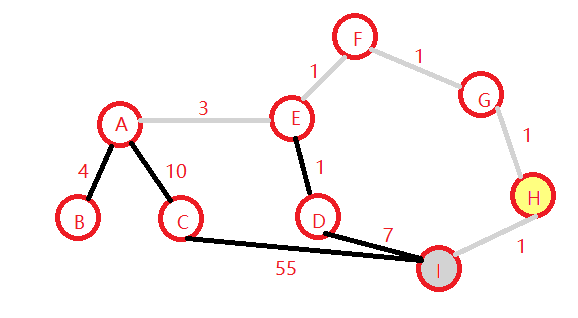1.2 实现它！

1.2.1 谈一谈我的想法：

1.2.2 需要的辅助的函数：

//根据两个顶点的位置 返回边的权
int getWeight(int srcindex,int destindex)
{
if (martix)
{
if (srcindex >= 0 && destindex >= 0 && srcindex < size && destindex < size)
{
return martix[srcindex][destindex];
}
}
}
//这个用于初始化从v开始到任意一个可以连接的顶点的权值
void dijkstra_setWeightSummary(int v, vector<int> &vec)
{
if (v >= 0 && v < size)
{
for (int u = 0; u < size; ++u)
{
//if (martix[v][u] == SAME)
//{
//	vec.push_back(NOEDGE);
//}
//else
//{
vec.push_back(martix[v][u]);
//}
}
}
}

1.2.3 这个是核心的函数

void do_dijkstra(int v0,vector<int> &Patharc,vector<int> &PathWeights)
{

vector<int> finalPath(size);//标志信息 保存前驱顶点
int v, w, k, min;//
//初始化数据 patharc保存的是从v0->vi的路径数组 pathweight表示的v0点有连线的顶点+权值
for (v = 0; v < size; ++v)
{
finalPath[v] = 0;
Patharc.push_back(0);
}
dijkstra_setWeightSummary(v0,PathWeights);//这个从起始点开始 吧从v0能到的点的边设置权值
PathWeights = 0;//出事的v0->v0的权为0
finalPath[v0] = 1;//这个表示v0这个路径被访问
for (v = 0; v < size; ++v)
{
min = NOEDGE;//这个是最小的权值 w为第一个可能的节点
for (w = 0; w < size; ++w)
{
if (!finalPath[w] && PathWeights[w] < min)
{
k = w;//这个k表示的就是第一个要被替换的位置（因为从V0到vw的权更小）
min = PathWeights[w];//保存这个最小的权值 继续比较找到更小的位置
}
}
finalPath[k] = 1;//目前找到的最小的权的目标点设为1 表示已经搜索了
for (w = 0; w < size; ++w)
{
if (!finalPath[w]/*如果是0的话 表示这个位置可以替换 如果是1的话表示这个位置之前已经定下来了*/ && (min + getWeight(k, w) < PathWeights[w]))
{
PathWeights[w] = min + getWeight(k, w);
Patharc[w] = k;//设置前驱节点 如果目标的权比之前得到地权要小的话。。更新那个最小权的点的前驱
}
}
}
//delete finalPath;
}

1.2.4 几个解释：
Patharc是用来保存前驱节点的数组
PathWeight这个是保存从V0到对应节点的最小的权的和对的数组
finalPath当finalPath[w]=1的时候表示已经求出了从V0->Vw的最短路径 这是一个标志
v用于循环中表示指定的一个顶点
min表示的是从Vw起始点到权最小的顶点的权值（注意不是累加顶点和）
k便是找到当min被赋值时所对应的Vw的下标
w是表示Vw的下标的循环变量

1.2.5 模拟计算：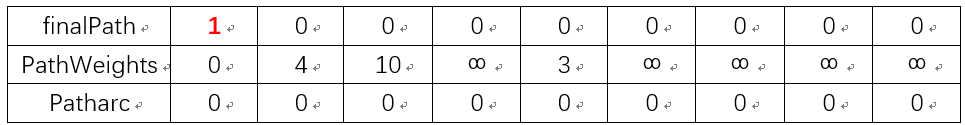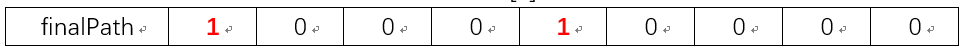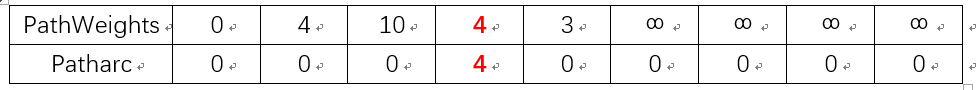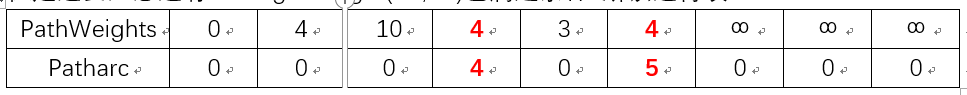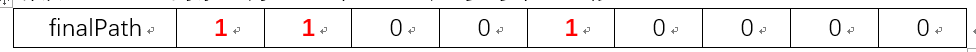min=4，k=3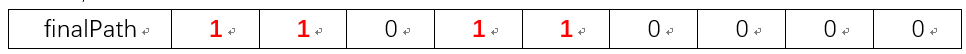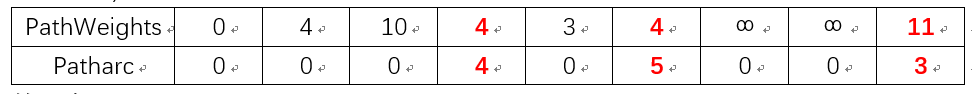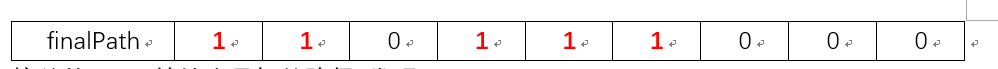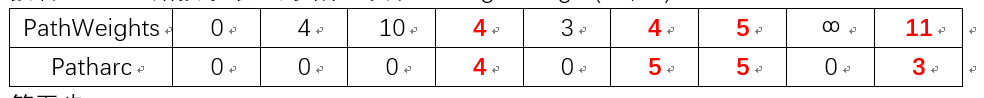v=5，min=5，k=6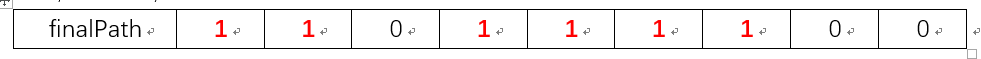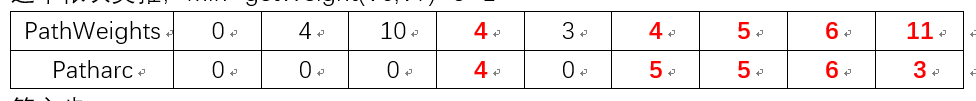v=6，min=6，k=1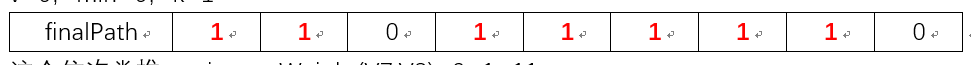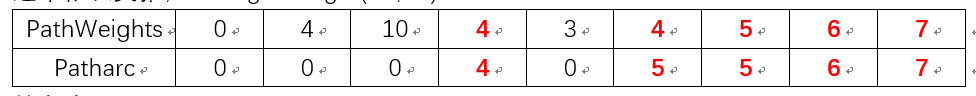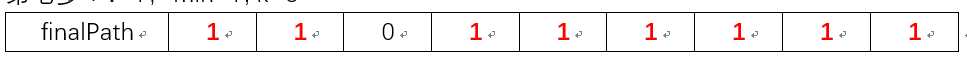v=8，min=10，k=2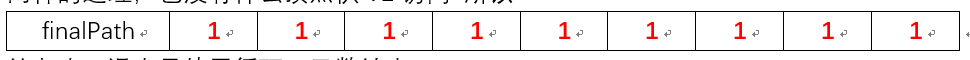1.1 Dijkstra的堆优化

void do_dijkstra_heap(int v0,vector<int> &pre,vector<int> &weight)
{
typedef std::pair<int, int> P;//P类型是保存从v0到该点second的最短的距离 每次从v0搜索路径的时候总是可以先进行搜索
std::priority_queue<P, std::vector<P>, std::greater<P>> que;//保存距离的最小堆.
weight[v0] = 0;//weight[i]是保存从v0出发到顶点为i的距离
que.push(P(0, v0));
while (!que.empty())
{
P p = que.top();
que.pop();//弹出
int v = p.second;//v是最短的点
if (p.first > weight[v])
continue;//如果这个边还要大 就不要再继续比较了 这个点就要不得，因为他是更新之前的从i->v的最大值（包括没有路径）
//定义weight[i]表示v0->i的距离 weight[v]=min(weight[v],weight[w]+weight(w->v))
for (int w = 0; w < size; ++w)
{
if (martix[v][w] != NOEDGE && martix[v][w] != SAME)//如果从v->w有捷径可走的话
{
int weight_vw = martix[v][w];
if (weight[w] > weight[v] + weight_vw)//v0->w 和v0->v(认为是最短的)->w哪一个更短?
{
weight[w] = weight[v] + weight_vw;
pre[w] = v;//前驱
que.push(P(weight[w], w));//把这个最小的权值放进去
}
}
}

}
}

2.Floyd算法

• 2.1 例子：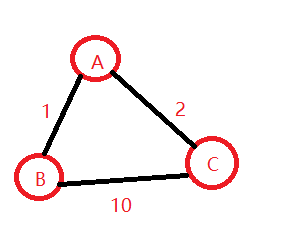索引 0 1 2 A: 0 0 1 2 B: 1 1 0 10 C: 2 2 10 0

 索引值 0 1 2 3 4 5 6 7 8 A: 0 0 4 10 ∞ 3 ∞ ∞ ∞ ∞ B: 1 4 0 ∞ ∞ ∞ ∞ ∞ ∞ ∞ C: 2 10 ∞ 0 ∞ ∞ ∞ ∞ ∞ 55 D: 3 ∞ ∞ ∞ 0 1 ∞ ∞ ∞ 7 E: 4 3 ∞ ∞ 1 0 1 ∞ ∞ ∞ F: 5 ∞ ∞ ∞ ∞ 1 0 1 ∞ ∞ G: 6 ∞ ∞ ∞ ∞ ∞ 1 0 1 ∞ H: 7 ∞ ∞ ∞ ∞ ∞ ∞ 1 0 1 I: 8 ∞ ∞ ∞ 55 7 ∞ ∞ 1 0

 索引值 0 1 2 3 4 5 6 7 8 A: 0 0 1 2 3 4 5 6 7 8 B: 1 0 1 2 3 4 5 6 7 8 C: 2 0 1 2 3 4 5 6 7 8 D: 3 0 1 2 3 4 5 6 7 8 E: 4 0 1 2 3 4 5 6 7 8 F: 5 0 1 2 3 4 5 6 7 8 G: 6 0 1 2 3 4 5 6 7 8 H: 7 0 1 2 3 4 5 6 7 8 I: 8 0 1 2 3 4 5 6 7 8

D-1[k][w]表示的是从Vk->Vw的权，D-1[v][w]表示的是从Vv->Vw的权。对于无向图，权D-1[w][v]= D-1[v][w]表示的就是Vw->Vv所以他们的权之和为Vk->Vv的权然后与Vk->Vv(直接)的权值之和比较。如果小的话，更新D-1[v][w]。注意我们讨论的是无向图，但是对于有向图的话，D-1[v][w]为∞（不会有那两个均不为∞能大于∞），这个∞总是会被替换的（其他路径），但是D-1[w][v]可能是一个别的值。仅仅是方向的区别。别忘了我们需要更新P数组的值。所以说白了所有的点需要从Vk中转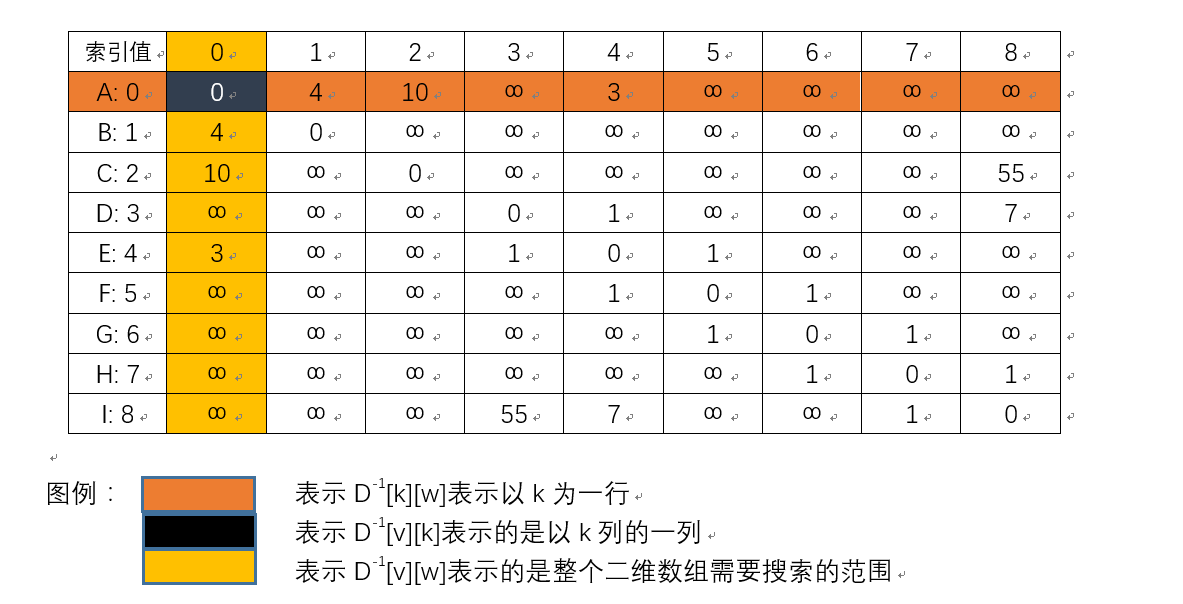//弗洛伊德算法
void do_floyd(vector<vector<int>> &P, vector<vector<int>> &D)
{
for (int i = 0; i < size; ++i)
{
dijkstra_setWeightSummary(i, D[i]);
for (int j = 0; j < size; ++j)
{
P[i].push_back(j);
}
}
int v, w, k;
//v是起始顶点左边 w是结束点坐标 k是中转点坐标 所有的顶点都会在Vk中转
for (k = 0; k < size; ++k)
{
for (v = 0; v < size; ++v)
{
for (w = 0; w < size; ++w)
{
if (D[v][w] > D[v][k] + D[k][w]) /*D[v][w] 表示的是整个二维数组需要搜索的范围 D[v][k]表示的是以k列的一列 D[k][w]表示以k为一行*/
{

D[v][w] = D[v][k] + D[k][w];
P[v][w] = P[v][k];
}
}
}
}
}

2.2 模拟计算：

D: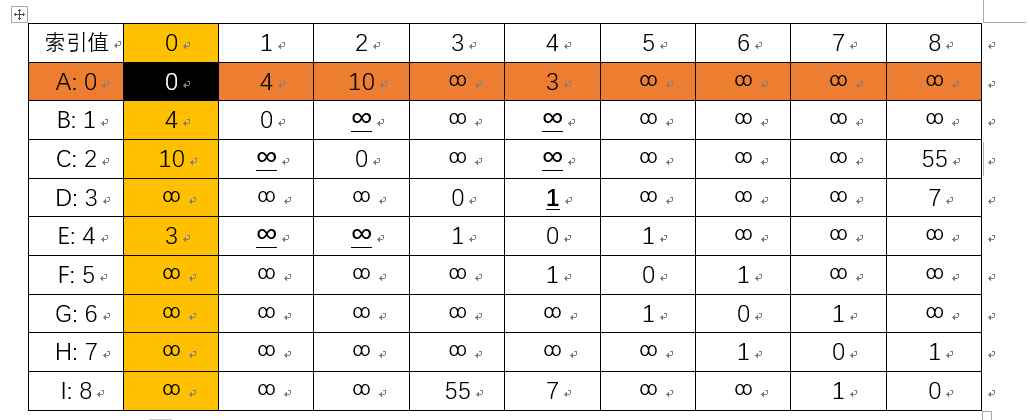D=∞而D+D=14
D=∞而D+D=4+3=7
D=∞而D+D=14
D=∞而D+D=13
D=∞而D+D=7
D=∞而D+D=13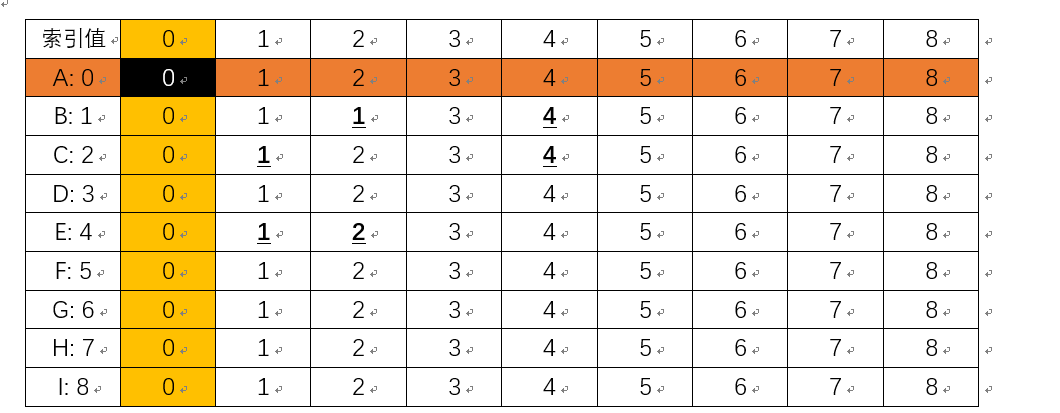P=P=0

P=P=0

P=0

P=0

P4]=0

P=0

 索引值 0 1 2 3 4 5 6 7 8 A: 0 0 4 10 4 3 4 5 6 7 B: 1 4 0 14 8 7 8 9 10 11 C: 2 10 14 0 14 13 14 15 16 17 D: 3 4 8 14 0 1 2 3 4 5 E: 4 3 7 13 1 0 1 2 3 4 F: 5 4 8 14 2 1 0 1 2 3 G: 6 5 9 15 3 2 1 0 1 2 H: 7 6 10 16 4 3 2 1 0 1 I: 8 7 11 17 5 4 3 2 1 0

P:

 索引值 0 1 2 3 4 5 6 7 8 A: 0 0 1 2 4 4 4 4 4 4 B: 1 0 1 0 0 0 0 0 0 0 C: 2 0 0 2 3 0 5 6 7 8 D: 3 4 4 4 3 4 4 4 4 4 E: 4 0 0 0 3 4 5 5 5 5 F: 5 4 4 4 4 4 5 6 6 6 G: 6 5 5 5 5 5 5 6 7 7 H: 7 6 6 6 6 6 6 6 7 8 I: 8 7 7 7 7 7 7 7 7 8

2.3 我们怎么获得路径呢？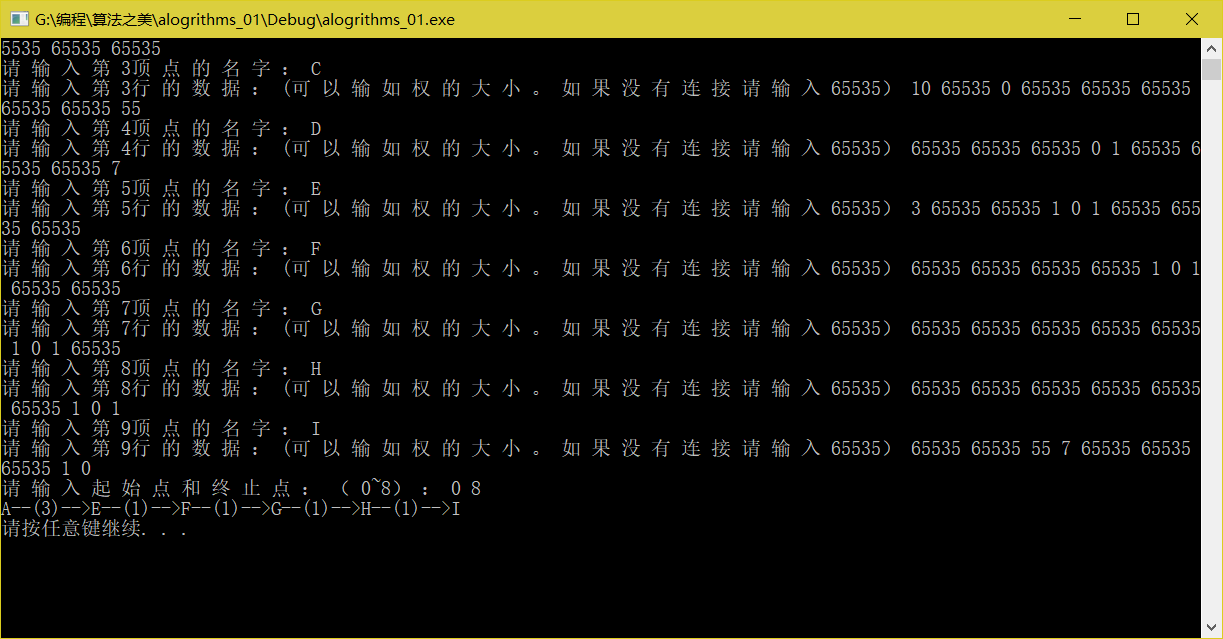3. 附上两个算法的类的接口：

void Find_MinPath_Dijkstra()
{
vector<int> arc, weight;
int v0;
cout << "请输入起始点：（0~" << size - 1 << "）：";
cin >> v0;
do_dijkstra(v0, arc, weight);
for (int i = 0; i < size; ++i)
{
if (i == v0)
continue;
std::cout << "从" << names[v0].name << "到" << names[i].name << "的最小的权为：" << weight[i] << ", 路径为："<<names[v0].name<<"->";
int vex = i;
std::stack<int> ids;
while (arc[vex] != v0)
{
ids.push(arc[vex]);
vex = arc[vex];//因为我每次加入的是前驱 所以只要vex与这arc[vex]是前驱关系就可以了
}
while (!ids.empty())
{
std::cout << names[ids.top()].name << "->";
ids.pop();
}
std::cout << names[i].name << std::endl;
}
}
void Find_MinPath_Floyd()
{
vector<vector<int>> arc(size), weight(size);
int v0,vx;
cout << "请输入起始点和终止点：（0~" << size - 1 << "）：";
cin >> v0>>vx;
do_floyd(arc, weight);
if (v0 >= 0 && v0 < size && vx >= 0 && vx < size)
{
int beg = v0;
int wei = v0;
while (beg != vx)
{
wei= arc[beg][vx];
cout << names[beg].name << "--("<<weight[beg][wei]<<")-->";
beg = wei;
}
//记得最后一个元素
cout << names[beg].name << endl;
}
}
void Find_MinPath_Dijkstra_Heap()//堆优化的Dijkstra算法
{
int v0;
std::cout << "请输入起点:";
std::cin >> v0;
std::vector<int> pre(size),weight(size,NOEDGE);
do_dijkstra_heap(v0, pre,weight);
//打印前驱
for (int i = 0; i < size; ++i)
{
if (i != v0)
{
std::stack<int> pathpoint;
pathpoint.push(i);
int k = i;
while (pre[k] != v0)
{
pathpoint.push(pre[k]);
k = pre[k];
}
pathpoint.push(v0);
while (!pathpoint.empty())
{
std::cout << names[pathpoint.top()].name;
pathpoint.pop();
if (!pathpoint.empty())
std::cout << "->";
}
std::cout << " min path weight : " << weight[i];
std::cout << std::endl;
}
}
}

4. 参考：

4.1 《大话数据结构》